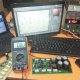Breaking News
Home / How to make an Amplifier? / How many voltage and amperes need for 2 transistor circuit board?

# How many voltage and amperes need for 2 transistor circuit board?

## How many voltages and amperes need for 2 transistor circuit board:

This is a 2 transistor circuit board. Here in this article, we can learn how to make transistor circuit diagram of 2sa1943 and 2sc5200. We can use 2 transistors in this circuit. one is 2sa1943 and another one is 2sc5200. How many voltages and amperes have to use? We know one transistor can take maximum 1.2 amperes so here in this circuit, we are using 2 transistors. We know Voltage X Ampere = Watts. we are using 2 transistors so 1.2X2=2.4. So we can use 2.4 amperes in this circuit. How many Watts can we get from this circuit? We can use 2.4 amperes. If we use 35-0-35 Voltage and 2.5-ampere transformer then we can get Voltage X ampere= Watts, So 35X2.5= 87.5 Watts. Please remember that Always use original or good quality one transistor. How to know about original transistor? there have many groups of the transistor in our market. For an example, 2sa5200 is the transistor number, and there also have another number, that is the Group number it starts from 120 to up 900++. Please always try to use up to 500++ Group number of the transistor.how to make transistor audio amplifier. How many voltages and amperes need for 2 transistor circuit board?

Here we can see 2 transistors. we can see the different group number. one is 120 and another one is 728. 120 number is the low category transistor. the good quality transistor is up to 500. here we can see 728 it’s the good one. the transformer is important for the amplifier. all transistor amplifier transistor need center tap transformer. we need positive, negative and ground voltage. here we can watch a video, so let’s go to watch the video

transistor circuit diagram of 2sa1943 and 2sc5200

Thanks a lot to be with us. If you want another post then please visit our website.

We have another post for you. like as repairing amplifier,